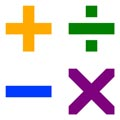# NYS CCLS Gr.4 Math Mod

• New York State Common Core Math CurriculumFourth grade mathematics is about (1) developing understanding and fluency with multi-digit multiplication, and developing understanding of dividing to find quotients involving multi-digit dividends; (2) developing an understanding of fraction equivalence, addition and subtraction of fractions with like denominators, and multiplication of fractions by whole numbers; and (3) understanding that geometric figures can be analyzed and classified based on their properties, such as having parallel sides, perpendicular sides, particular angle measures, and symmetry.

Curriculum modules in mathematics are marked by in-depth focus on fewer topics. They integrate the CCLS, rigorous classroom reasoning, extended classroom time devoted to practice and reflection through extensive problem sets, and high expectations for mastery. The time required to complete a curriculum module will depend on the scope and difficulty of the mathematical content that is the focus of the module.

Sequence of Grade 4 Modules Aligned with the Standards

Module 1: Place Value, Rounding, and Algorithms for Addition and Subtraction

Module 2: Unit Conversions and Problem Solving with Metric Measurement

Module 3: Multi-Digit Multiplication and Division

Module 4: Angle Measure and Plane Figures

Module 5: Fraction Equivalence, Ordering, and Operations

Module 6: Decimal Fractions

Module 7: Exploring Multiplication

 Key Areas of Focus for 3-5: Multiplication and division of whole numbers and fractions—concepts, skills, and problem solving Required Fluency: 4.NBT.4 Add and subtract within 1,000,000.

Annotated 3-8 ELA and Mathematics State Test Questions

https://www.engageny.org/resource/new-york-state-common-core-sample-questions

# Related Files

•# Grade 4 CCLS Math Curriculum Guide - Bflo Pub Schools

•# Grade 4 - Math Module 1 - Topics A-E & Assessments

•# Grade 4 - Math Module 2 - Topics A-B & Assessments

•# Grade 4 - Math Module 3 - Topics A-H & Assessments

•# Grade 4 - Math Module 4 - Topics A-D & Assessments

•# Grade 4 - Math Module 5 - Topics A-H & Assessments

•# Grade 4 - Math Module 6 - Topics A-E & Assessments

•